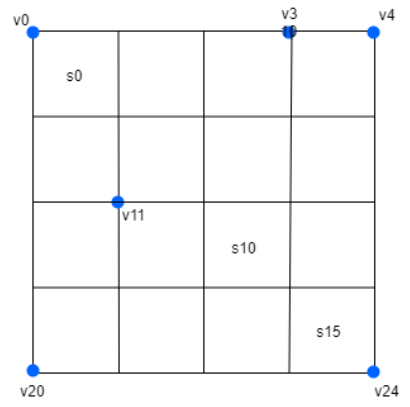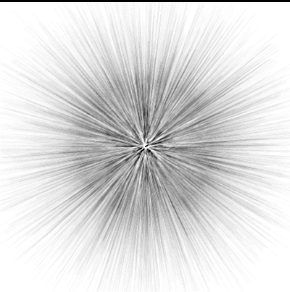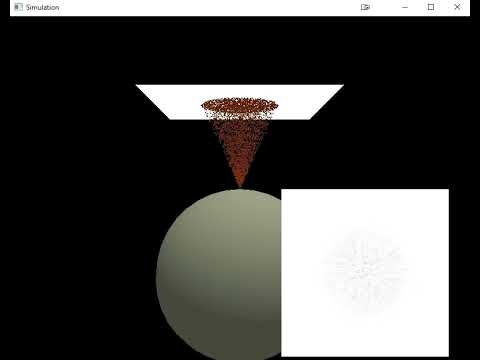# Rendering a plane divided into sectors of different colors

I want to draw a square plane that is divided into smaller square sectors of different colors.
The plane is made up of sectors and vertices, as in the figure below:The above plane is divided onto NxN sectors. N=4 in this example, hence we have 16 sectors (I have labelled only 3 in figure to make it clearer).

We also have 25 vertices in the plane.

Now: suppose a large number of balls hit our plane. If a ball hits one of the sectors, we add the number 1 to this sector value. When we count the ball hits, we get a 2D array representing the values assigned to each sector. For example like this one:

0 18 60 0
10 8979 8613 25
20 8229 9304 44
0 48 28 0

It can be seen that the balls mainly hit the centre of the plane.

The resulting numbers represent the colors of each sector, but we need to normalise them beforehand to represent values between 0 and 1. Let’s assume that 0 represents the color white and 10 000 represents the color black (R=G=B). By dividing our numbers by 10 000 we get the normalised values. However, in OpenGL we have to assign the colors to the vertices, so we need to somehow transfer the colors from the sectors to the vertices (we will normalise them after that):

We create a 2D array to represent the vertices colors, its dimensions will be (N+1)x(N+1).

The values of the vertices are obtained by calculating the average value from the values of the sectors that a vertex connects.

• If the vertex lies on a corner of the plane, we divide the value from the sector it touches and divide by 4.
• If a vertex connects only two sectors, we sum their values and divide by 4.

The result of the above is the following 2D array of colors assigned to the vertices:

0 4.5 19.5 15 0
2.5 2251.75 4417.5 2174.5 6.25
7.5 4309.5 8781.25 4496.5 17.25
5 2074.25 4402.25 2344 11
0 12 19 7 0

Let’s check one of the vertices, to be sure, that the above values are correct. Let’s consider the value 2251.75, which belongs to v6 vertex (according to figure at the beginning of this post). Vertex v6 connects four sectors: s0, s1, s4, and s5. The values of these sectors are: 0, 18, 10 and 8979. The sum of these numbers is 9007. We divide it by 4, which gives 2251.75. This is the value assigned to our vertex v6.

We divide values from the array by 10 000 to normalise them. We obtain:

0 0.00045 0.00195 0.0015 0
0.00025 0.225175 0.44175 0.21745 0.000625
0.00075 0.43095 0.878125 0.44965 0.001725
0.0005 0.207425 0.440225 0.2344 0.0011
0 0.0012 0.0019 0.0007 0

The next step is to substract above values from 1, as our plane is white at the beginning and it gets darker when balls hit it. The results is:

1 0.99955 0.99805 0.9985 1
0.99975 0.774825 0.55825 0.78255 0.999375
0.99925 0.56905 0.121875 0.55035 0.998275
0.9995 0.792575 0.559775 0.7656 0.9989
1 0.9988 0.9981 0.9993 1

The last step is to send above values to GPU using glBufferSubData and after rendering we obtain:It is difficult to evaluate the correctness of the results obtained if our N is so small. Hence, I have increased N to 400, and the result is given below:You can see straight lines that look like sunbeams. This does not look like the distribution we should get on our plane.

Below is the video of the balls hitting the plane:To sum up:
We got the correct color values at the vertices. Then they were sent to the graphics card and the plane was rendered. What we got does not appear to be the correct result. Maybe there is something wrong with the rendering method?

My initRenderData() method is given below:

``````void SpriteRenderer_plane_sectors::initRenderData()
{
// positions and colors
float xPos=-0.5f, yPos=0.5f, zPos=0.0f; // initialization, we start from left top corner

for (size_t y = 0; y < rows + 1; ++y)
{
for (size_t x = 0; x < columns + 1; ++x)
{
// positions
positions.push_back(xPos + static_cast<float>(x) / columns);
positions.push_back(yPos - static_cast<float>(y) / rows);
positions.push_back(zPos);

// colors
colors.push_back(1.0f);
colors.push_back(1.0f);
colors.push_back(1.0f);
}
}

// indices
for (size_t y = 0; y < rows; ++y)
{
for (size_t x = 0; x < columns; ++x)
{
indices.push_back(y * (columns + 1) + x);
indices.push_back(y * (columns + 1) + x + 1);
indices.push_back(y * (columns + 1) + x + 1 + rows);

indices.push_back(y * (columns + 1) + x + 1);
indices.push_back(y * (columns + 1) + x + 1 + rows);
indices.push_back(y * (columns + 1) + x + 1 + rows + 1);
}
}

glGenVertexArrays(1, &VAO);
glBindVertexArray(VAO);

glGenBuffers(1, &VBO);
glBindBuffer(GL_ARRAY_BUFFER, VBO);
glBufferData(GL_ARRAY_BUFFER, sizeof(float) * (positions.size() + colors.size()), NULL, GL_DYNAMIC_DRAW);

glBufferSubData(GL_ARRAY_BUFFER, 0, sizeof(float) * positions.size(), positions.data());
glBufferSubData(GL_ARRAY_BUFFER, sizeof(float) * positions.size(), sizeof(float) * colors.size(), colors.data());

glVertexAttribPointer(0, 3, GL_FLOAT, GL_FALSE, 3 * sizeof(float), (void*)0);
glVertexAttribPointer(1, 3, GL_FLOAT, GL_FALSE, 3 * sizeof(float), (void*)(sizeof(float) * positions.size()));

glGenBuffers(1, &EBO);
glBindBuffer(GL_ELEMENT_ARRAY_BUFFER, EBO);
glBufferData(GL_ELEMENT_ARRAY_BUFFER, sizeof(unsigned int) * indices.size(), indices.data(), GL_STATIC_DRAW);

glEnableVertexAttribArray(0); //0 = location
glEnableVertexAttribArray(1); //1 = colors

glBindVertexArray(0);
}
``````

My drawSprite() method:

``````void SpriteRenderer_plane_sectors::drawSprite(glm::vec3 position, glm::vec3 size, float rotate, int** array2D)
{

glm::mat4 projection = glm::perspective(glm::radians(camera.Zoom), static_cast<float>(SCR_WIDTH) / static_cast<float>(SCR_HEIGHT), 0.1f, 100.0f);

glm::mat4 view = camera.GetViewMatrix();

glm::mat4 model = glm::mat4(1.0f);
model = glm::translate(model, position);

model = glm::translate(model, glm::vec3(0.5f * size.x, 0.5f * size.y, 0.5f * size.z)); // move origin of rotation to center of quad
model = glm::rotate(model, glm::radians(rotate), glm::vec3(1.0f, 0.0f, 0.0f)); // then rotate
model = glm::translate(model, glm::vec3(-0.5f * size.x, -0.5f * size.y, -0.5f * size.z)); // move origin back

model = glm::scale(model, glm::vec3(size));

convert2DArrayToObtainColors(array2D);

glBindBuffer(GL_ARRAY_BUFFER, VBO);
glBufferSubData(GL_ARRAY_BUFFER, sizeof(float) * positions.size(), sizeof(float) * colors.size(), colors.data());

glBindVertexArray(VAO);

//glPolygonMode(GL_FRONT_AND_BACK, GL_LINE);
//glDrawArrays(GL_TRIANGLES, 0, 36);
glDrawElements(GL_TRIANGLES, indices.size(), GL_UNSIGNED_INT, 0);
glBindVertexArray(0);
}
``````

And my convert2DarrayToObtainColors() method (it uses out 2D sectors data to obtain 2D vertices data):

``````void SpriteRenderer_plane_sectors::convert2DArrayToObtainColors(int** sectors_array2D)
{
// Vertices array was created in a constructor
// Assiging the vertices values:
for (size_t i = 0; i < rows + 1; ++i) // iterate through 2D vertices array
{
for (size_t j = 0; j < columns + 1; ++j)
{
float sectors_sum = 0.0f;
if (i < rows && j < columns) // adding value of bottom right (from the vertex) sector
sectors_sum += sectors_array2D[i][j];
if (i > 0 && j > 0) // adding value of top left (from the vertex) sector
sectors_sum += sectors_array2D[i - 1][j - 1];
if (i > 0 && j < columns) // adding value of top right (from the vertex) sector
sectors_sum += sectors_array2D[i - 1][j];
if (i < rows && j > 0) // adding value of bottom left (from the vertex) sector
sectors_sum += sectors_array2D[i][j - 1];
sectors_sum /= 4;
vertices_array2D[i][j] = sectors_sum;

// we substract from 1, because we want to get black color. We also normalize our value:
float normalized_value = 1 - (vertices_array2D[i][j] / highest_sector_value);
// We have to be sure, that the result won't be lower then zero. If it is lower, we replace it by 0
if (normalized_value <= 0.0f)
vertices_array2D[i][j] = 0.0f;
else
vertices_array2D[i][j] = normalized_value;

// Converting our 2D array to 1D array. Later it will be used to construct our colors vector
vertices_array1D[i * (columns + 1) + j] = vertices_array2D[i][j];
}
}

// Saving colors to colors vector:
int i = 0;
for (size_t color_index = 0; color_index < colors.size(); color_index+=3)
{
colors[color_index] = vertices_array1D[i];
colors[color_index + 1] = vertices_array1D[i];
colors[color_index + 2] = vertices_array1D[i];
++i;
}
}
``````

Problem solved.
It turned out that the 2D array representing the values assigned to each sector was causing the problem.
I was sure, that I have correct values in that array, but I was wrong.
The element values in the array were incremented more than once for each photon, and the photons continued to move after reaching the height of the plane, even though their motion was not rendered (position were changed). Further photon positions were written to the array, which is why such a strange distribution was obtained.

This topic was automatically closed 183 days after the last reply. New replies are no longer allowed.